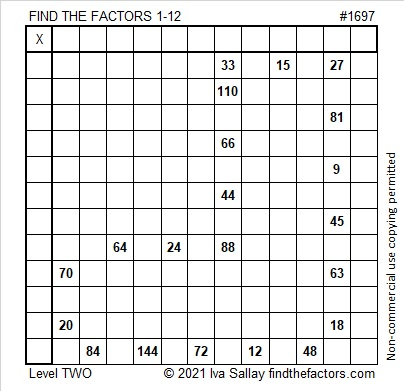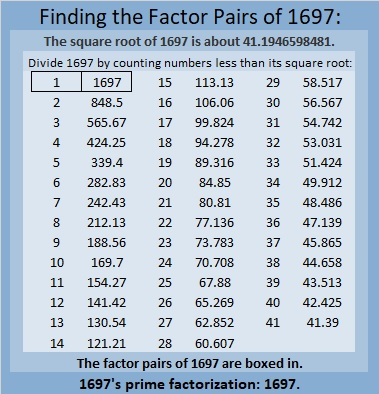# 1697 A Boot in the Window

Contents

### Today’s Puzzle:

Tonight throughout many parts of the world children will place their polished boots in a window awaiting a visit from St. Nick. In the morning they will find their boots filled with favorite candies.

You can solve this boot puzzle by writing the numbers from 1 to 12 in both the first column and the top row so that those numbers and the given clues will become the start of a multiplication table.### Factors of 1697:

• 1697 is a prime number.
• Prime factorization: 1697 is prime.
• 1697 has no exponents greater than 1 in its prime factorization, so √1697 cannot be simplified.
• The exponent in the prime factorization is 1. Adding one to that exponent we get (1 + 1) = 2. Therefore 1697 has exactly 2 factors.
• The factors of 1697 are outlined with their factor pair partners in the graphic below.How do we know that 1697 is a prime number? If 1697 were not a prime number, then it would be divisible by at least one prime number less than or equal to √1697. Since 1697 cannot be divided evenly by 2, 3, 5, 7, 11, 13, 17, 19, 23, 29, 31, 37, or 41, we know that 1697 is a prime number.

### More About the Number 1697:

1697 is the sum of two squares:
41² + 4² = 1697.

1697 is the hypotenuse of a Pythagorean triple:
328-1665-1697, calculated from 2(41)(4), 41² – 4², 41² + 4².

Here’s another way we know that 1697 is a prime number: Since its last two digits divided by 4 leave a remainder of 1, and 41² + 4² = 1697 with 41 and 4 having no common prime factors, 1697 will be prime unless it is divisible by a prime number Pythagorean triple hypotenuse less than or equal to √1697. Since 1697 is not divisible by 5, 13, 17, 29, 37, or 41, we know that 1697 is a prime number.

This site uses Akismet to reduce spam. Learn how your comment data is processed.